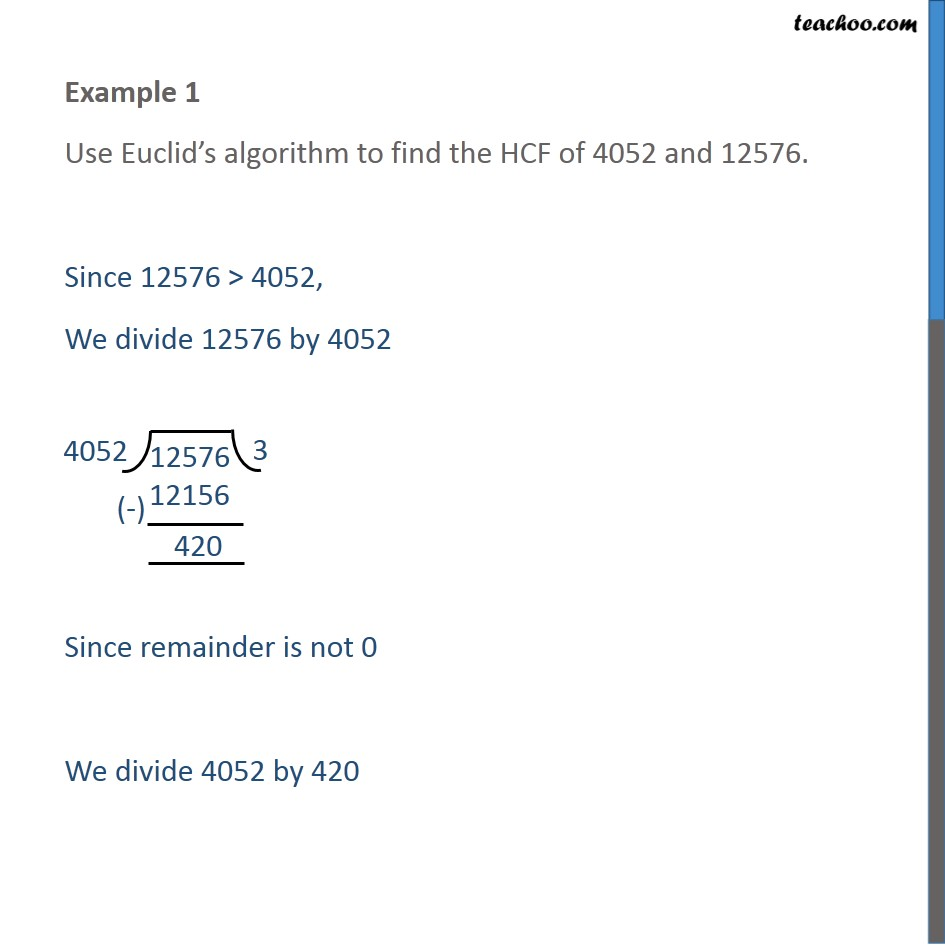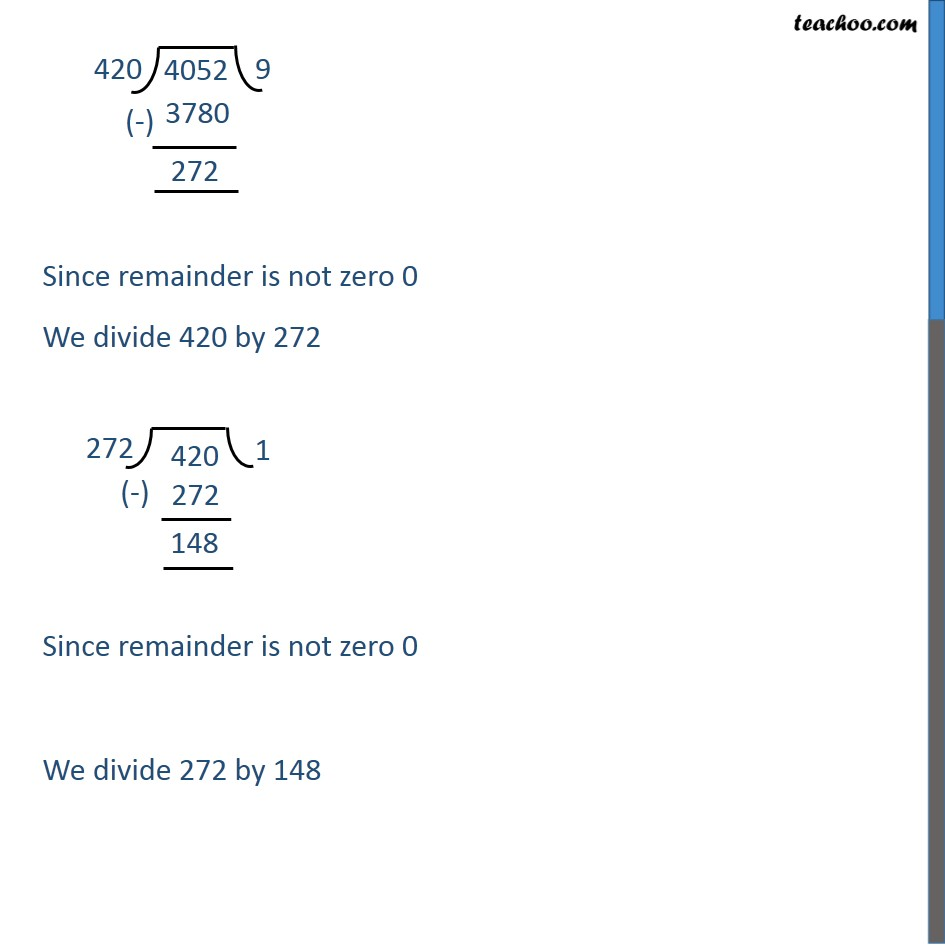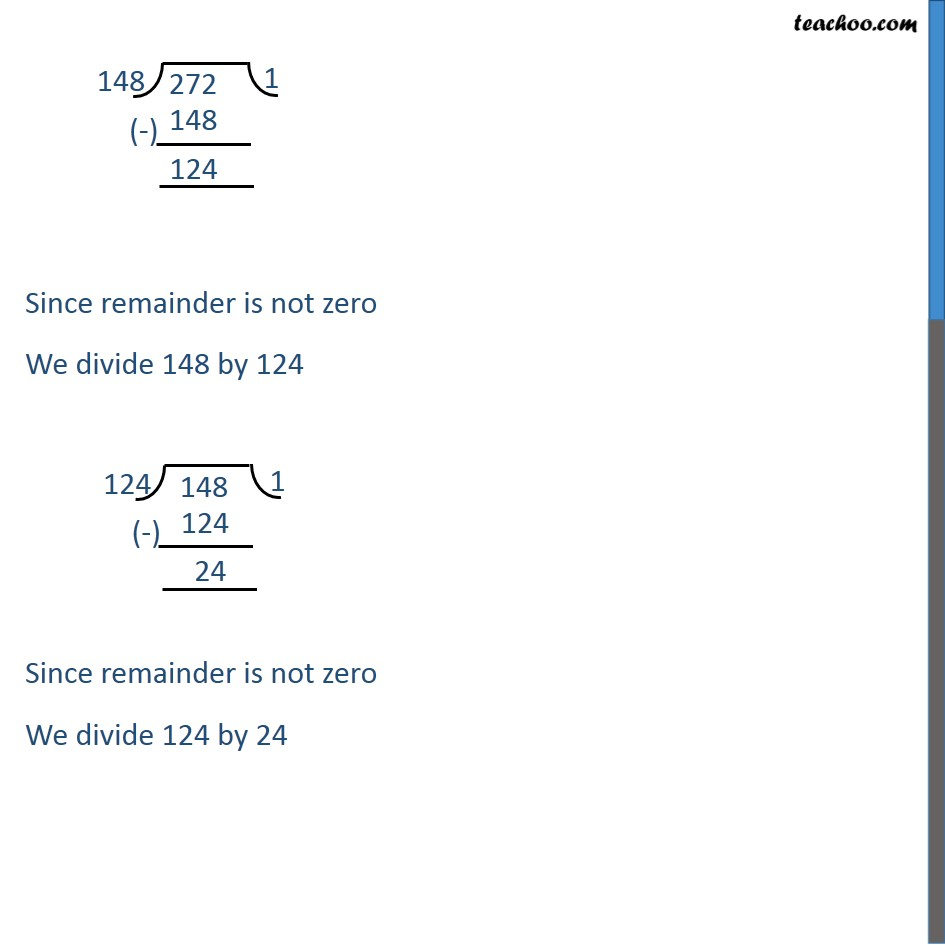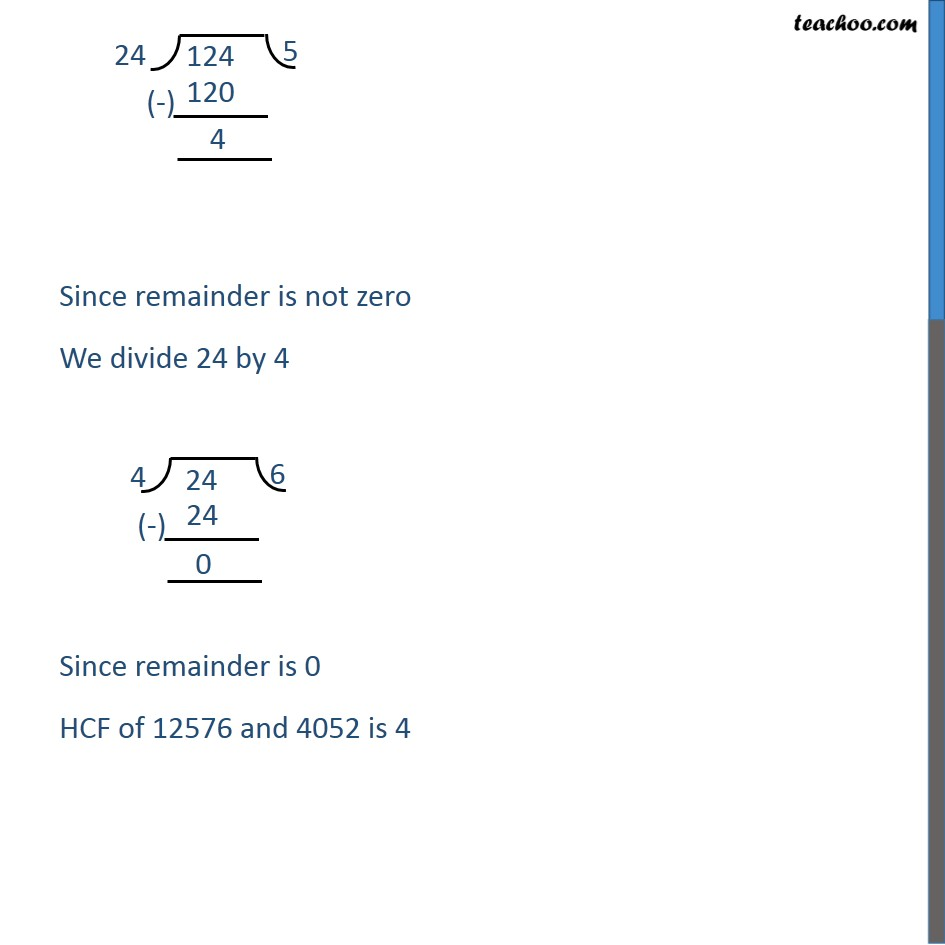Check Important Questions and Chapter Summary -https://you.tube/Real-Numbers-Class-101. Chapter 1 Class 10 Real Numbers
2. Concept wise
3. HCF using Euclid's Division Algorithm

Transcript

Example 1 Use Euclid’s algorithm to find the HCF of 4052 and 12576. Since 12576 > 4052, We divide 12576 by 4052 Since remainder is not 0 We divide 4052 by 420 Since remainder is not zero 0 We divide 420 by 272 Since remainder is not zero 0 We divide 272 by 148 Since remainder is not zero We divide 148 by 124 Since remainder is not zero We divide 124 by 24 Since remainder is not zero We divide 24 by 4 Since remainder is 0 HCF of 12576 and 4052 is 4

HCF using Euclid's Division Algorithm